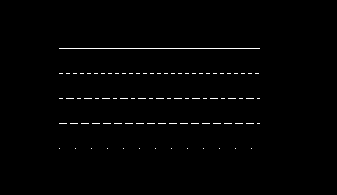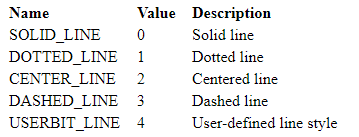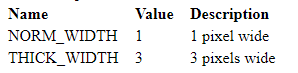Open in App
Not now

# setlinestyle() function in C

• Difficulty Level : Medium
• Last Updated : 25 Jan, 2018

The header file graphics.h contains setlinestyle() function which sets the style for all lines drawn by line, lineto, rectangle, drawpoly, and so on.

Syntax :

```void setlinestyle(int linestyle, unsigned upattern,
int thickness);
```

Examples :

```Input : x = 200, y = 100
Output :x and y are initialized as (200, 100).
For every line, value of y increments
by 25 to change the position.
The line style keep changing corresponding
to value of first parameter(c).
```

Explanation : linestyle specifies in which of several styles subsequent lines will be drawn (such as solid, dotted, centered, dashed).upattern is a 16-bit pattern that applies only if linestyle is USERBIT_LINE (4). In that case, whenever a bit in the pattern word is 1, the corresponding pixel in the line is drawn in the current drawing color. A value for ‘upattern’ must always be supplied. It is simply ignored if ‘linestyle’ is not USERBIT_LINE (4).
thickness specifies whether the width of subsequent lines drawn will be normal or thick.Below is the implementation of setlinestyle() function :

 `// C Implementation for setlinestyle()``#include `` ` `// driver code``int` `main()``{``    ``// gm is Graphics mode which is``    ``// a computer display mode that``    ``// generates image using pixels.``    ``// DETECT is a macro defined in``    ``// "graphics.h" header file``    ``int` `gd = DETECT, gm;`` ` `    ``// variable to change the``    ``// line styles``    ``int` `c;`` ` `    ``// initial coordinate to ``    ``// draw line``    ``int` `x = 200, y = 100;`` ` `    ``// initgraph initializes the``    ``// graphics system by loading a``    ``// graphics driver from disk``    ``initgraph(&gd, &gm, ``""``);`` ` `    ``// To keep track of lines``    ``for` `( c = 0 ; c < 5 ; c++ )``   ``{``       ``// setlinestyle function``       ``setlinestyle(c, 0, 1);`` ` `       ``// Drawing line``       ``line(x, y, x+200, y);``       ``y = y + 25;``   ``}`` ` `    ``getch();`` ` `    ``// closegraph function closes the``    ``// graphics mode and deallocates``    ``// all memory allocated by``    ``// graphics system .``    ``closegraph();`` ` `    ``return` `0;``}`

Output :

``````

My Personal Notes arrow_drop_up